Drawbacks of sequential storage

1. Fixed size of memory allocated:

Static implementation means allocating fixed amount of memory of design time. We cannot increase or shrink the memory at run time. If memory utilized at run time is less than allocated then it will waste the memory. If more memory is required than allocated, it cannot be increased at run time.

1. Inefficient memory utilization:

As static implementation allocates fixed amount of memory, we cannot increase or shrink the memory at run time. Therefore, memory utilization is not efficient.

1. Insertion and deletion operations are difficult:

Insert and delete operations performed on linear data structure is in sequential manner. If insertion or deletion is at middle position then we have to shift the data by one position, which is complicated. It will take more processing time. Linked lists allow insertion and removal of nodes at any point in the list, with a constant number of operations.

There is a special data structure called as linked list that overcomes the drawbacks of sequential storage. Linked list provides the flexibility to allocate memory at run time means the size of memory can be increased or decreased at run time.

Memory is allocated at each run time, it will not be contiguous. Hence, In order to keep the elements related the address of next element should be stored along with each element, so that they can be accessed. This type of list is called as Linked list.

#### Definition:

A linked list is an ordered collection of elements called nodes, linked with another item. Each node has two parts, first part contains the information field and second part contains the address of the next node. The address part of last node of linked list will have NULL value.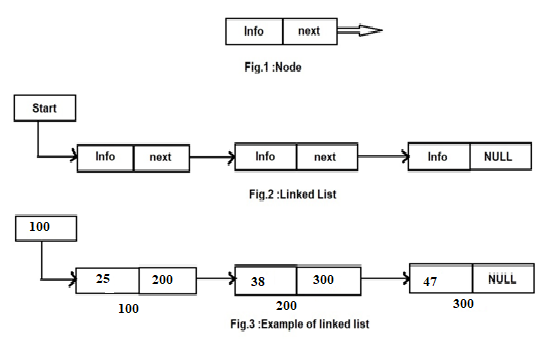Linked list are of following types

This type, the element are organized in a linear fashion and last node contains a NULL value.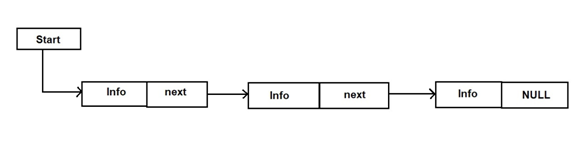This type, linked list contains two pointers; previous- pointing to the previous node and next- pointing to the next node.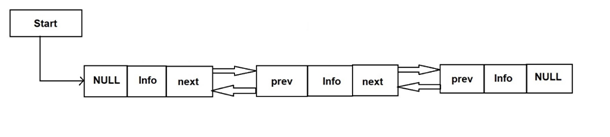In this type, the last node contains the address of first node instead of NULL pointer.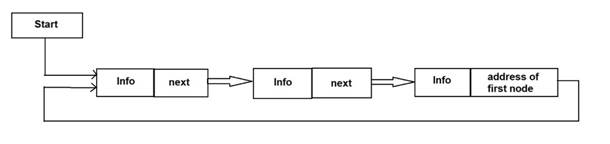In this type, the last node contains the address of first node instead of NULL pointer and the first node contains the address of last node.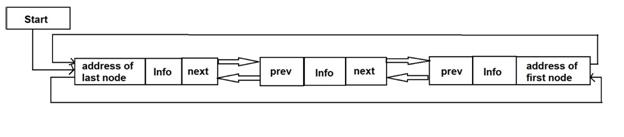The Linear linked list can be implemented using self-referential structure. It can represent in memory with following declaration:

struct node

{

int data;

struct node *next ;

};

typedef struct node NODE ;

NODE *start;

The doubly linked list can be implemented using self-referential structure. It can represent in memory with following declaration:

struct node

{

int data;

struct node *prev, *next ;

};

typedef struct node NODE ;

NODE *start ;

Allocating memory and assigning values to node

N1 and N2 are two nodes to be created. Link these two nodes. The list will look like as follows: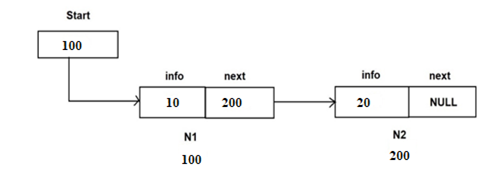struct node *N1, *N2, *start;

N1 = (struct node *) malloc(sizeof(struct node));

N1 -> info = 10 ;

Start = N1;

N2 = (struct node *) malloc(sizeof(struct node));

N2 -> info = 20 ;

N1 ->next = N2 ;

N2 -> next = NULL ;

The above code will create two nodes, which are connected to each other as shown in figure.

#### Some More:

POP- Introduction to Programming Using ‘C’

DS – Data structure Using C

OOP – Object Oriented Programming

Java Programming

DBMS – Database Management System

RDBMS – Relational Database Management System